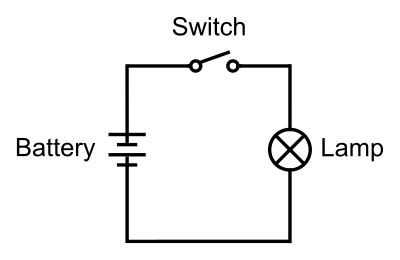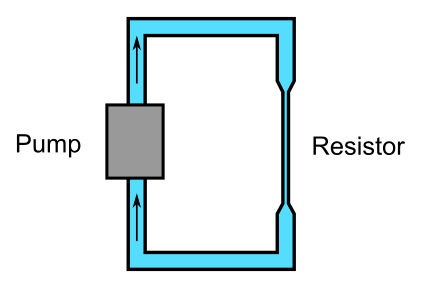# Ohm's law

Martin McBride, 2020-07-05
Tags battery lamp switch ohms law
Categories electronics electricity

In this section we will look at Ohm's law. We will start with the simple electric circuit we looked at previously:To recap, basic operation of this circuit is:

• The battery creates a voltage.
• The voltage causes a flow of electrons round the circuit, called an electric current.

There is one further aspect of this we haven't discussed:

• The light bulb creates a resistance to the flow of electricity.

## Resistance

Resistance can be thought of as a bit like friction. If you try to push a heavy box along a rough floor, it will be hard work - it takes work (energy) to move the box.

The same thing happens with electrical resistance. The light bulb resists the flow of electricity, and it is the voltage from the battery that provides the energy to force the electricity through the bulb.

In both cases, the energy supplied gets converted mainly into heat. The heavy box won't actually get hot, because quite a small amount of energy is spread across the whole base of the box. In the case of a light bulb, the electrical energy is dissipated in a short length of very thin wire, which gets so hot it glows brightly.

## Units

Voltage is measured in volts, of course, usually represented by the letter V. It is named after Italian physicist Alessandro Volta.

Electric current is measured in amperes, often shortened to amps, usually represented by the letter A. It is named after French physicist André-Marie Ampère.

Resistance is measured in Ohms, usually represented by the Greek letter Ω (Omega), or the letter R on systems that do not support Greek characters. It is named after German physicist Georg Simon Ohm. The choice of the letter Omega is pure wordplay, based on the similar sounding names.

## Ohm's law

Ohm's law can be state as the current flowing through a circuit is equal to the voltage divided by the resistance of the circuit:

A = V/R


Where A is the current in amps, V is the voltage in volts, and R is the resistance in ohms. The current that flows through a resistor depends linearly on the voltage applied (if you double the voltage, twice as much current will flow). The current is inversely proportional to the resistance (if you double the resistance, the current will halve).

The formula can be rearranged like this:

R = V/A
V = R.A


## Characteristics of different materials

Many metals conduct electricity very well. Silver has the highest conductivity (ie lowest resistance) of any metal, followed by copper, and then gold. Copper is normally used as it is by far the cheapest of the three. Reasonably thick copper wire has such low resistance that it is normally assumed to be zero in most electronic circuits.

For any particular metal, the thinner he wire, the greater the resistance. Also, as you might imagine, a longer length of the same wire will have greater resistance.

Many materials don't conduct electricity at all under normal circumstances, and are called insulators. Plastic and air are typical examples.

There are other materials that can conduct electricity, but have a much higher resistance than metals. These materials include carbon, and various metal oxides, and metals that contain impurities.

## Resistors

In electronics we often use components called resistors. A resistor has a specific, known resistance. You can get resistors with values that are anywhere from a few ohms right up to millions of ohms. Resistors are usually made of a thin film of conductive material - carbon, metal oxide or pure metal, depending on the desired resistance.

Resistors are used to control currents and voltages in a circuit. Here is an example:A light emitting diode (LED) is a component that lights up when current flows through it. But unlike an incandescent bulb, and LED doesn't have much resistance of its own. If you connected it straight to a battery, a large current would flow, and the LED would burn out almost immediately.

If we place a resistor, R1, in series with the LED, it limits the current so that the LED lights up without burning out. The value of the resistor depends on the battery voltage, and the type of LED.

## Water analogy

Here is how a water "resistor" might work. The normal pipe is very wide and allows water to flow freely. But the resistor is a narrow section of pipe that restricts the flow of water.In this circuit, the pressure of the pump represents the voltage of the battery, the total water flow (in litres per second) is like the current, and the restriction of the pipe represents the resistor. Just like the electric circuit, a more powerful pump causes more water flow, whereas a narrower pipe causes less water flow.